0

# 图卷积和消息传递理论的可视化详解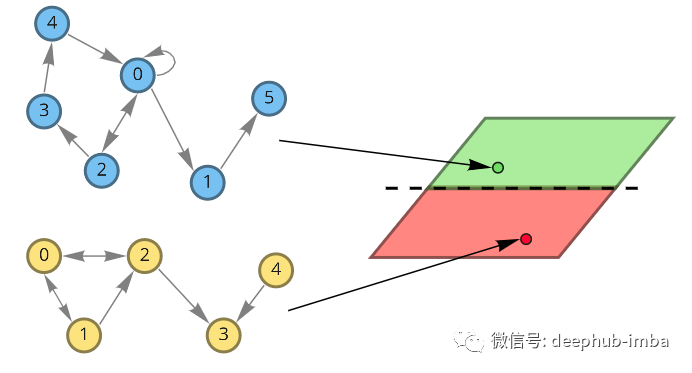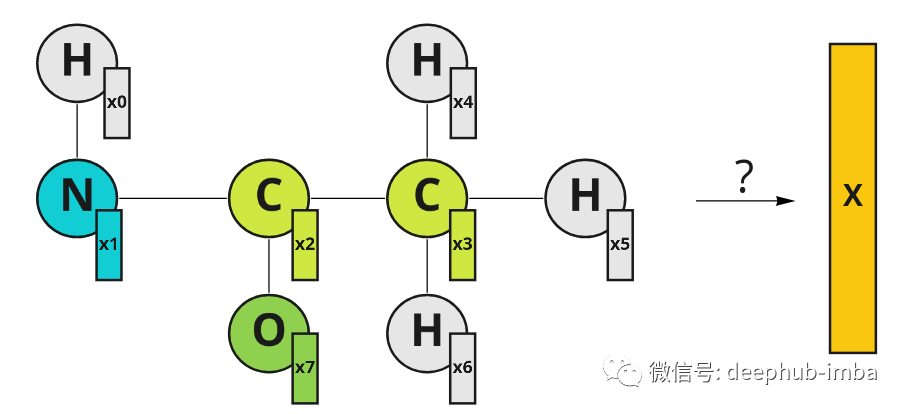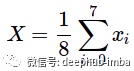## 图卷积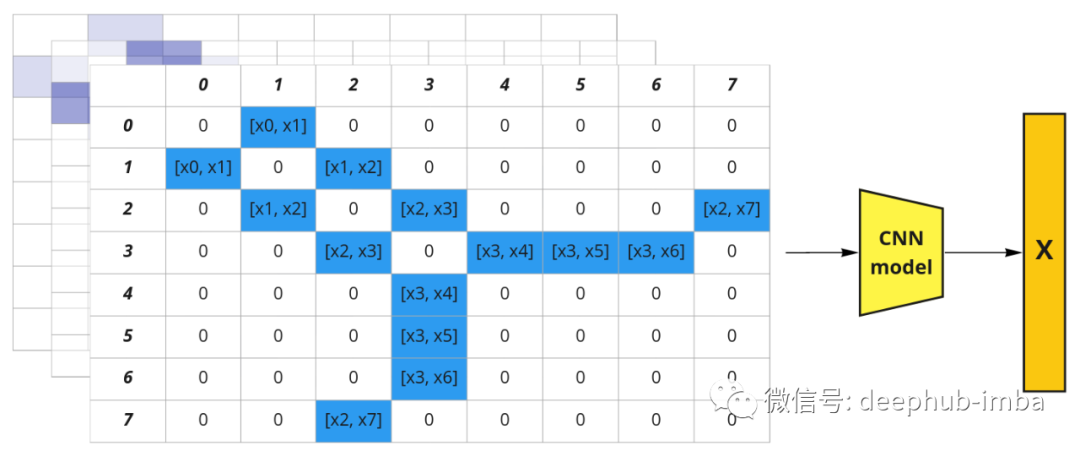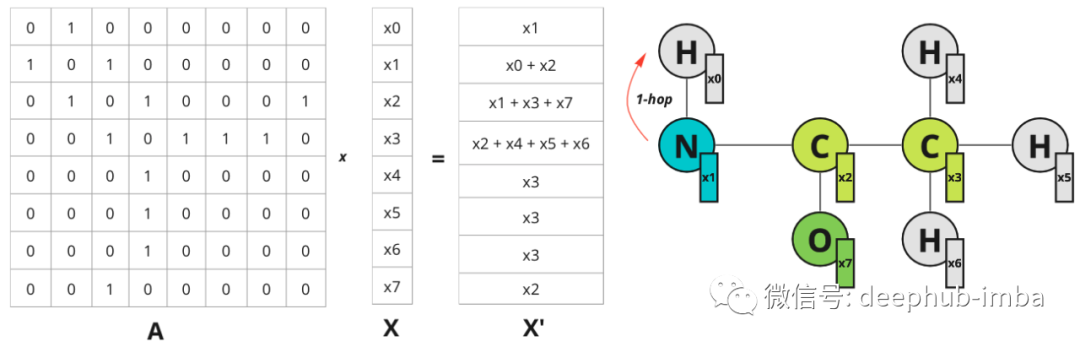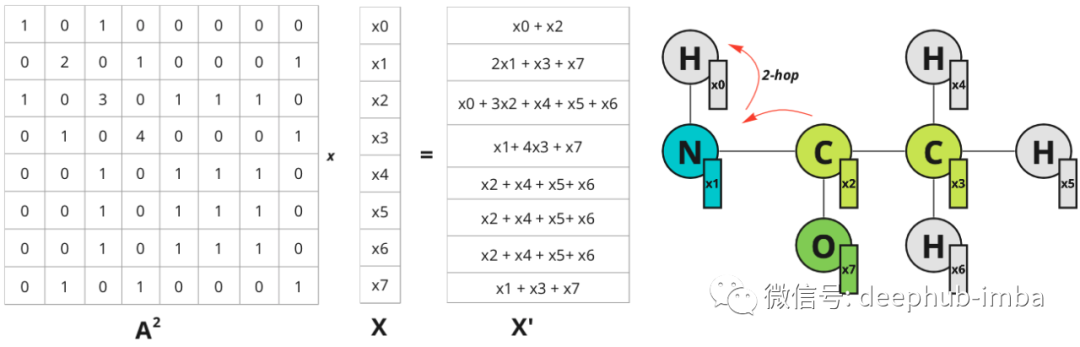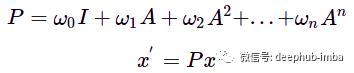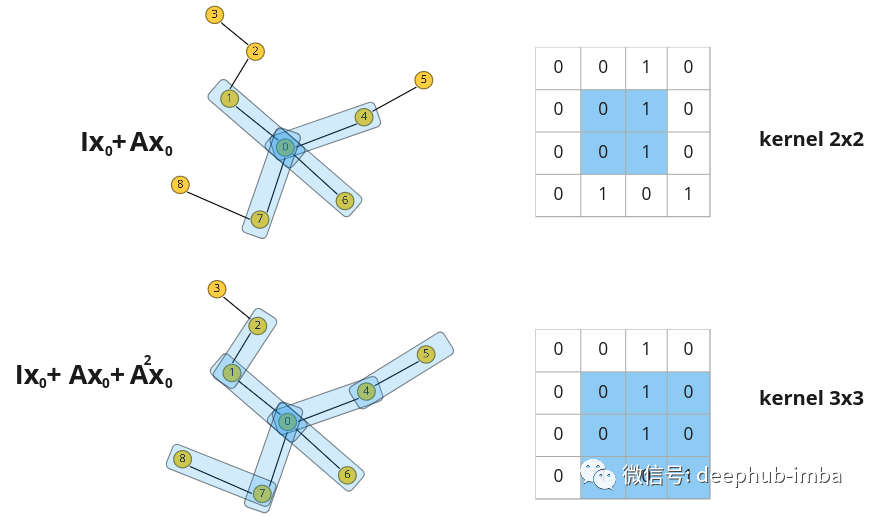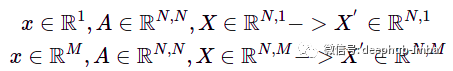x——节点特征向量，X——堆叠节点特征，M——节点特征向量的维度，N——节点数量。

## 消息传递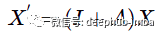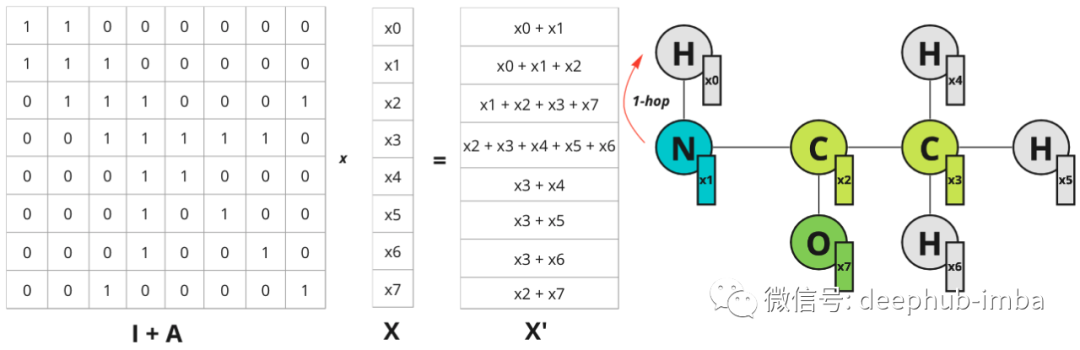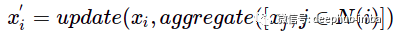N(i) 表示节点 i 的一跳距离邻居。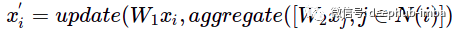W1——更新节点特征的权重矩阵，W2——更新相邻节点特征的权重矩阵。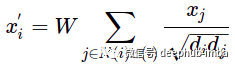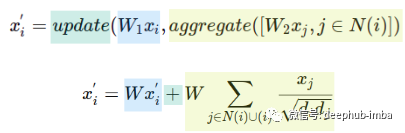## 一个实际的例子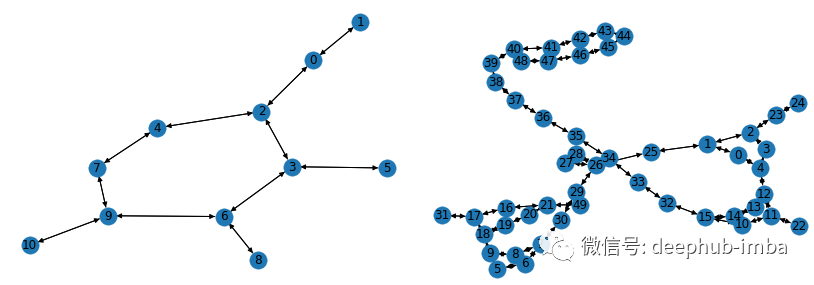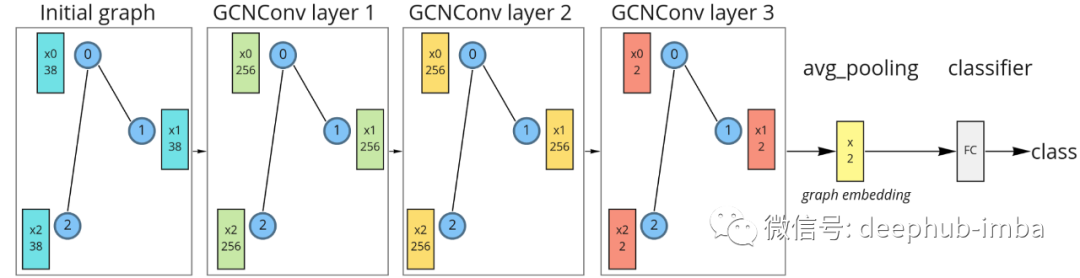``````from torch import nn
from torch.nn import functional as F
from torch_geometric.nn import GCNConv
from torch_geometric.nn import global_mean_pool

class GCNModel(nn.Module):
def __init__(self, feature_node_dim=38, num_classes=2, hidden_dim=256, out_dim=2):
super(GCNModel, self).__init__()
torch.manual_seed(123)
self.conv1 = GCNConv(feature_node_dim, hidden_dim)
self.conv2 = GCNConv(hidden_dim, hidden_dim)
self.conv3 = GCNConv(hidden_dim, out_dim)
self.linear = nn.Linear(out_dim, num_classes)

def forward(self, x, edge_index, batch):

# Graph convolutions with nonlinearity:
x = self.conv1(x, edge_index)
x = F.relu(x)
x = self.conv2(x, edge_index)
x = F.relu(x)
x = self.conv3(x, edge_index)

# Graph embedding:
x_embed = global_mean_pool(x, batch)

# Linear classifier:
x = self.linear(x_embed)

return x, x_embed
``````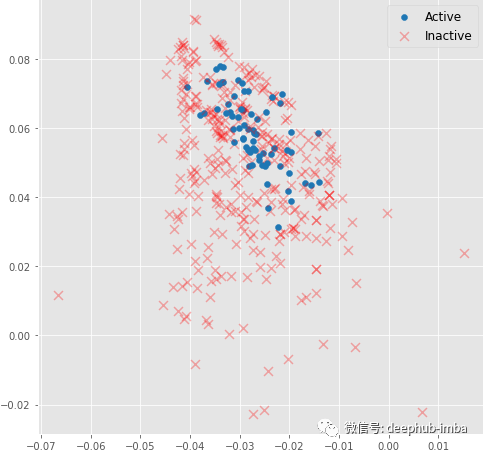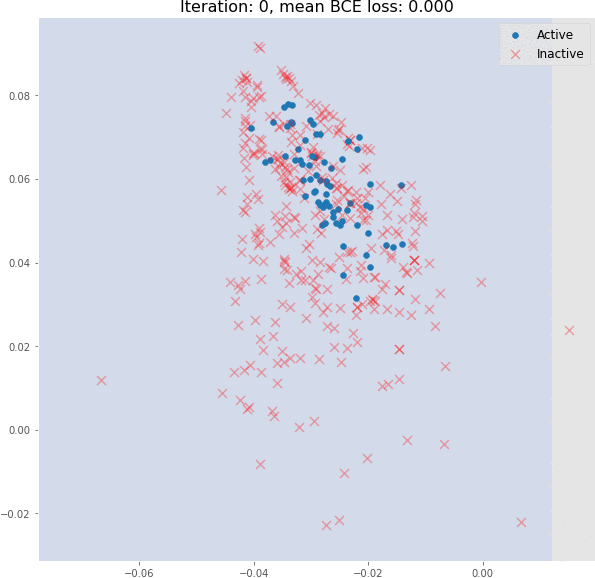## 总结

### “图卷积和消息传递理论的可视化详解”的评论:

##### 关于作者##### Deephub